# How-to | Build missing partitions with a scenario¶

Consider the following situation:

• You have a Dataiku Flow with datasets partitioned by date (format: YYYY-MM-DD)

• You run daily a scenario to build this Flow with the current date

You’d like to have a way to build your flow/scenario for (many) dates other than the current date, in particular for all missing dates/partitions.

You can easily adapt this how-to to other similar use cases with partitions.

## Step 1: Scenario to build your Flow for one given partition¶

You have a Dataiku Flow with datasets partitioned by date that you would like to rebuild.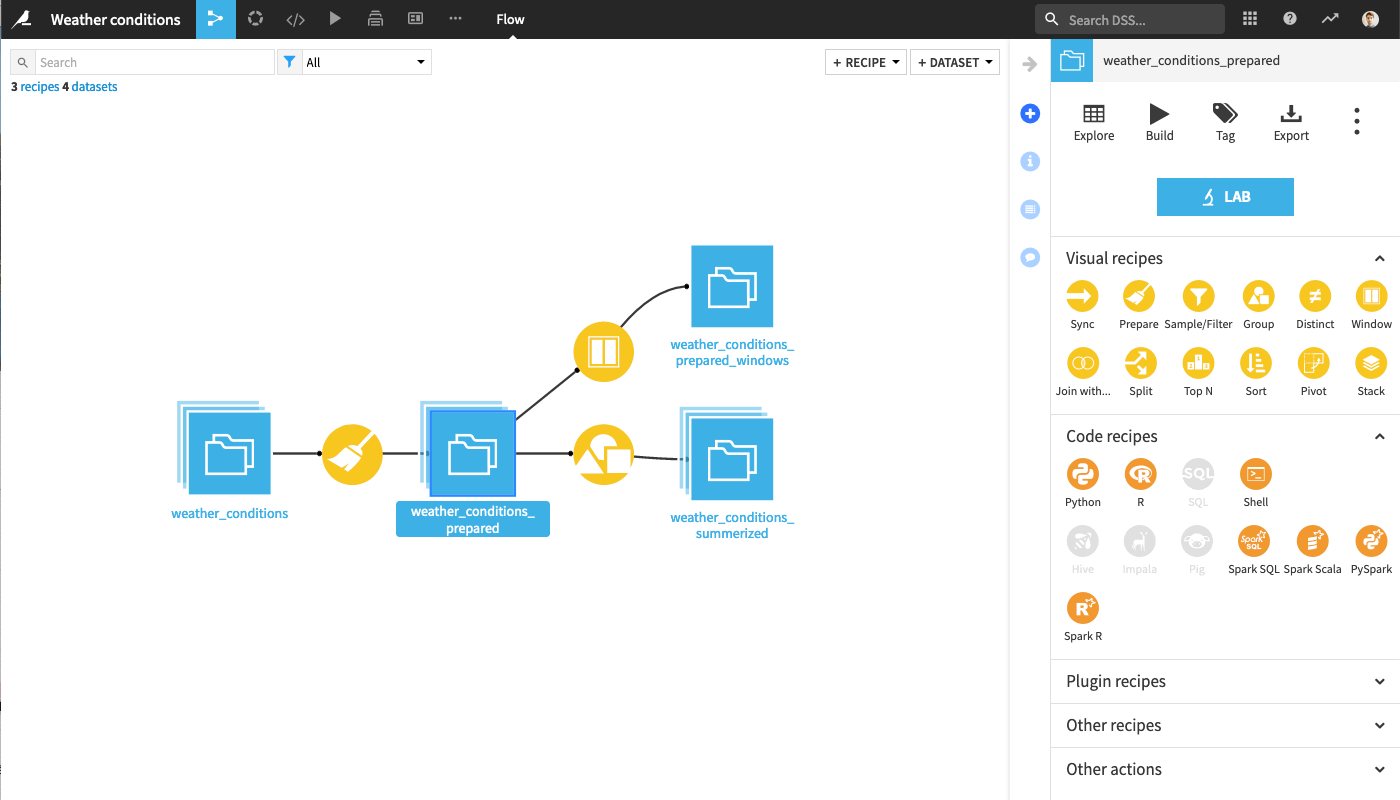First, define a scenario that runs the Flow for a single partition.

You probably already have such a scenario that runs, for example, for the current day using the keyword CURRENT_DAY as partition identifier.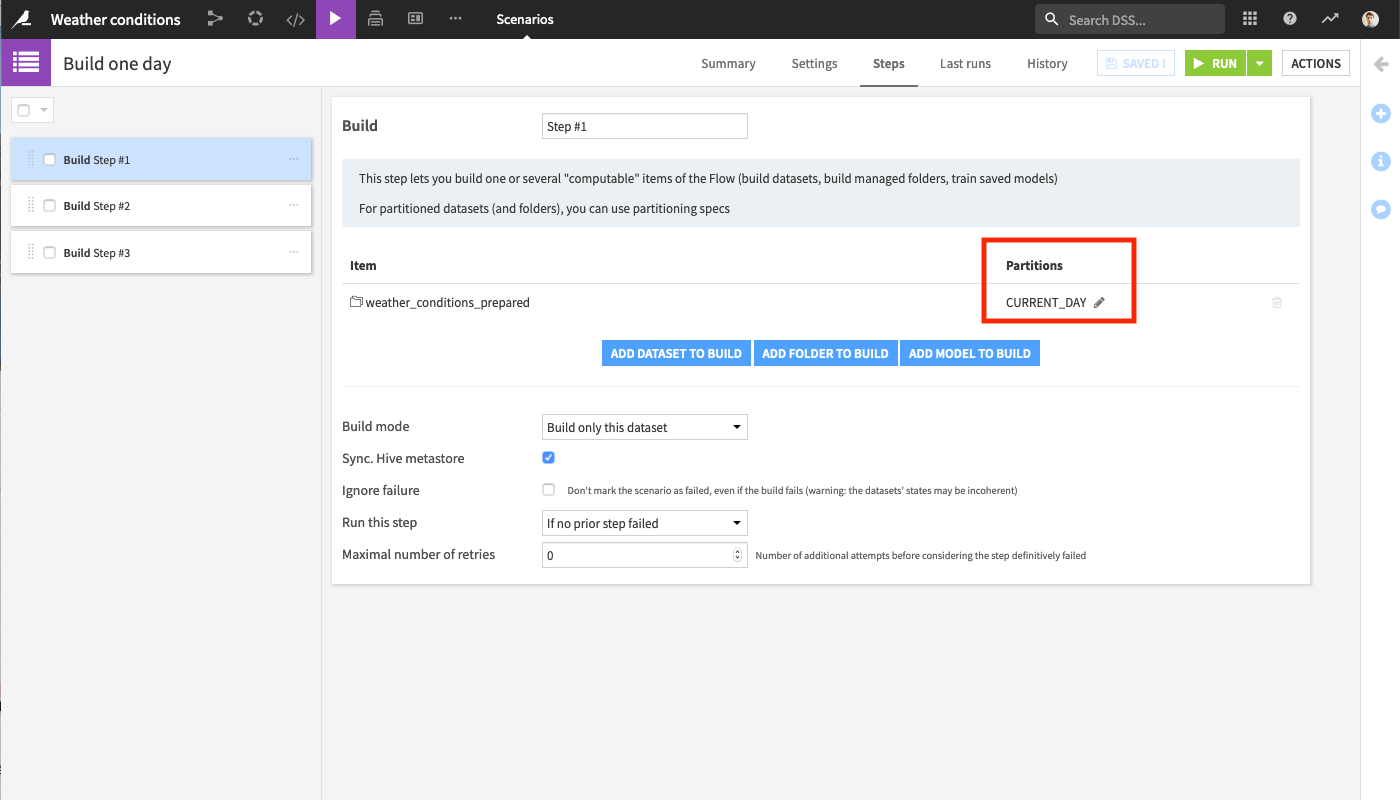Add a new step in your scenario that will first run to define a scenario variable.

Let’s call this variable partition and evaluate it with the following Dataiku formula:

coalesce(partition_to_build, scenarioTriggerParam_partition_to_build, now().toString('yyyy-MM-dd'))


This variable either gets the value of another variable called partition_to_build if defined (that our main scenario will define in step 2), the value of scenarioTriggerParam_partition_to_build that we can define manually, or the current date as a fallback.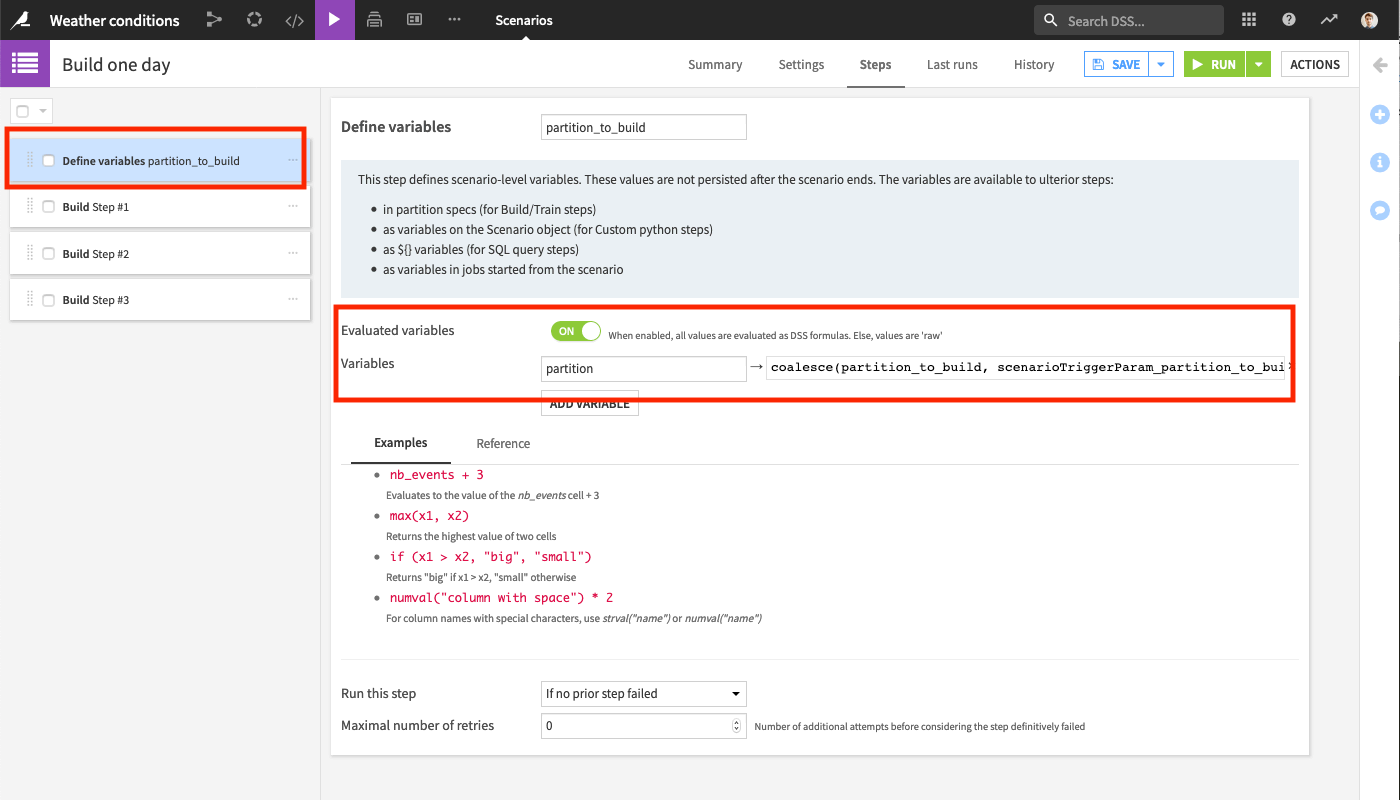Now, use this variable in the build steps as a partition identifier:

\${partition}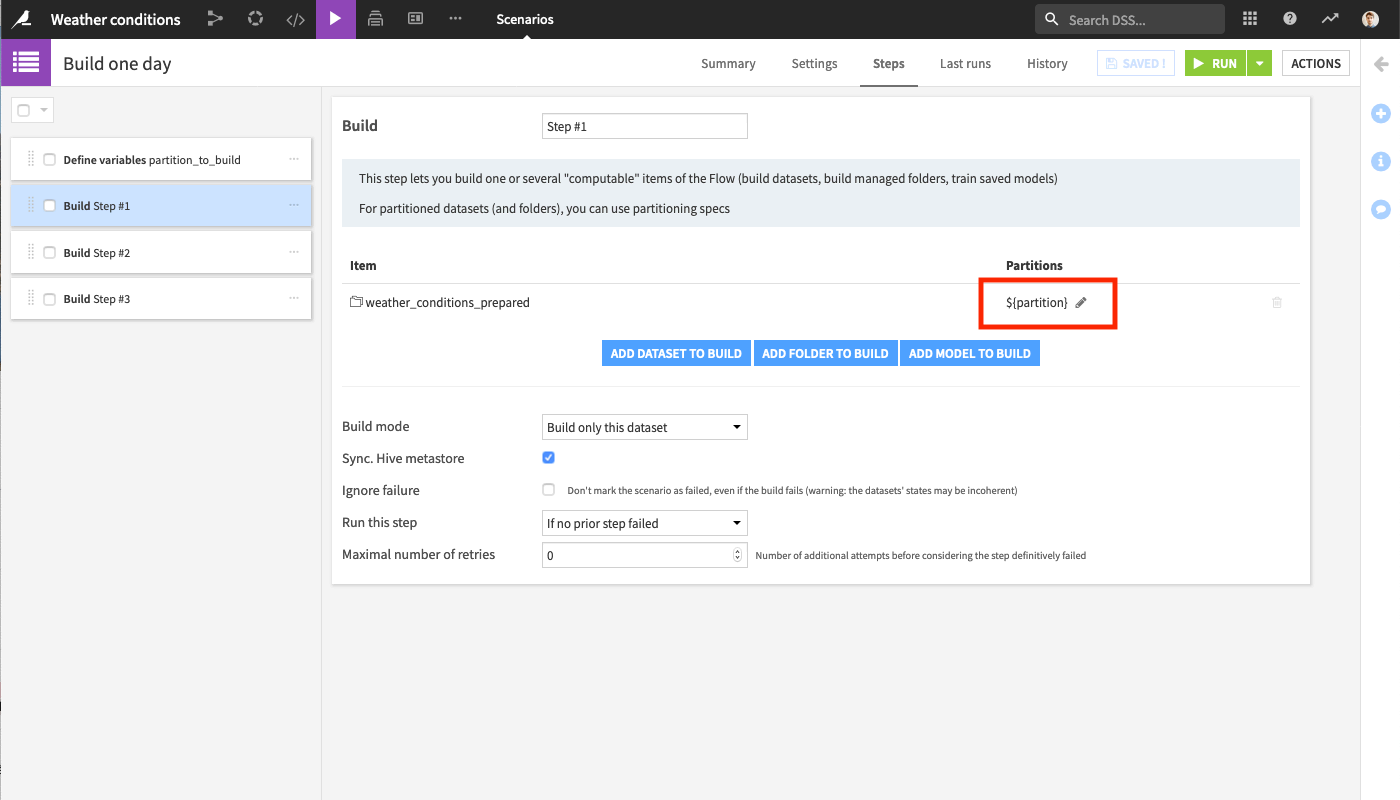You can try to run the scenario. It will run for the current day.

You can also run the scenario for another date choosing the “Run with custom parameters” in the top-right corner and entering a value for the parameter “partition_to_build”: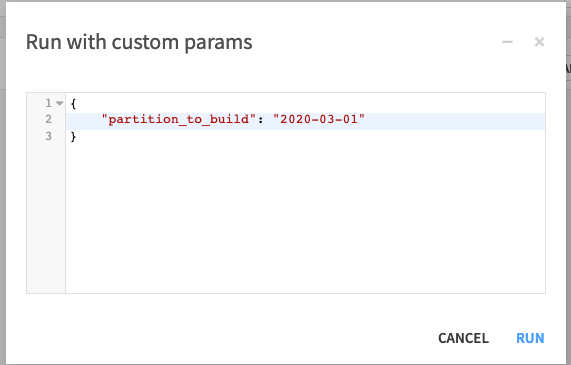## Step 2: Meta-scenario that runs the first scenario for all missing partitions¶

Now that we have a scenario that can build the Flow for a given partition, let’s create another scenario that will be able to run this scenario for all missing partitions.

First, create a “Custom Python script” scenario.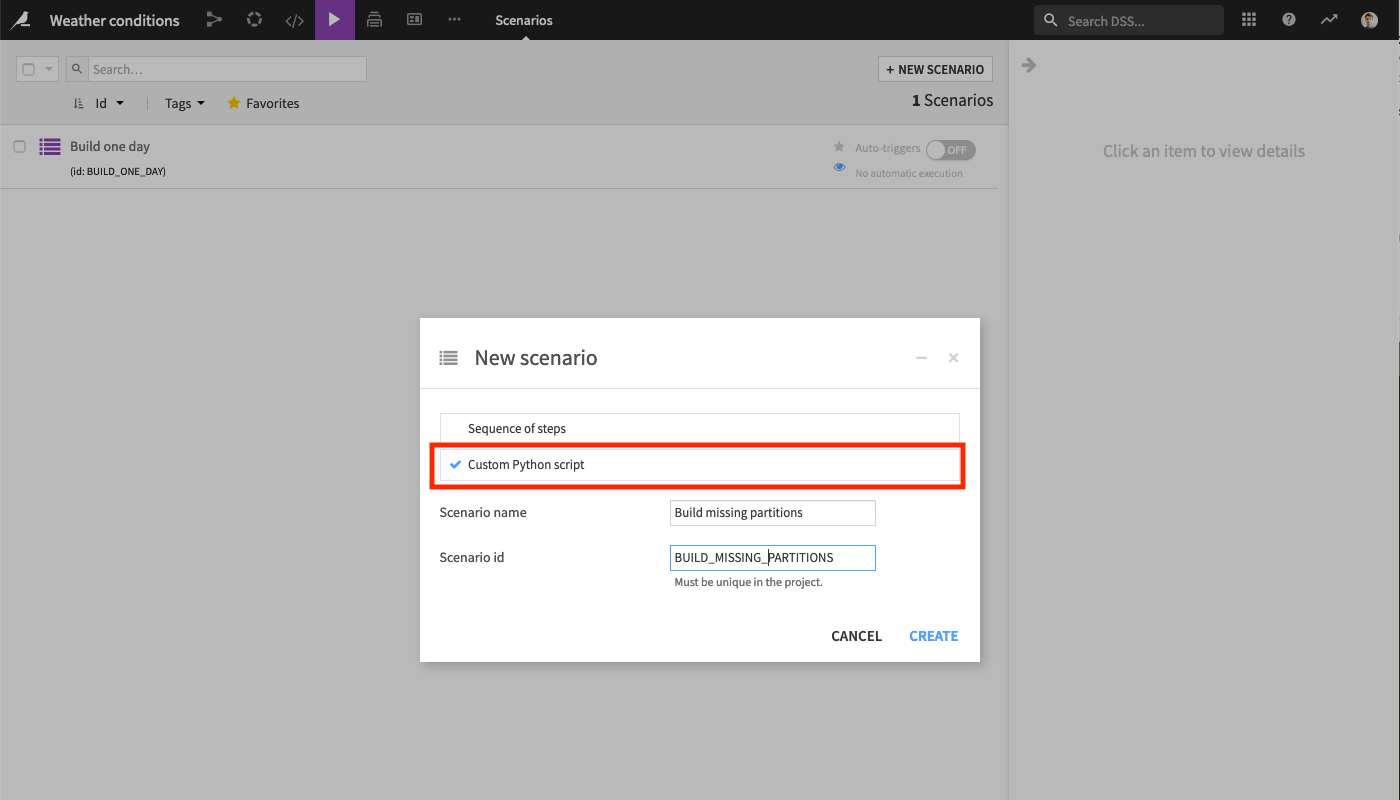You can now add a script that:

• gets all existing partitions

• generates a list of partitions that should exist

• finds missing partitions (difference of the two following lists)

• executes the scenario to build the Flow for any missing partition, one by one

from dataiku.scenario import Scenario
import dataiku
from datetime import timedelta, date

# object for this scenario
scenario = Scenario()

# let's get all curent existing partitions from a dataset of the flow
dataset = dataiku.Dataset('weather_conditions_prepared')
partitions = dataset.list_partitions()
print("Existing partitions:")
print(partitions)

# generate all partitions that should be buikt (here from Jan 1st 2020 until current day)
def dates_range(date1, date2):
for n in range(int ((date2 - date1).days)+1):
yield date1 + timedelta(n)
all_dates = [dt.strftime("%Y-%m-%d") for dt in dates_range(date(2020, 1, 1), date.today())]
print("Partitions that should exist:")
print(all_dates)

# let's find missing partitions
for partition in all_dates:
if partition not in partitions:
print("%s : missing partition" % partition)
# let's set a variable (on the current scenario) with the missing partition to build
scenario.set_scenario_variables(
partition_to_build=partition
)
# let's run the scenario that builds the flow for a given partition
# note that scenario variables are propagated to children scenarios, so the scenario
# will be able to read the variable 'partition_to_build'
scenario.run_scenario("BUILD_ONE_DAY")


Here is the same Python script as a scenario.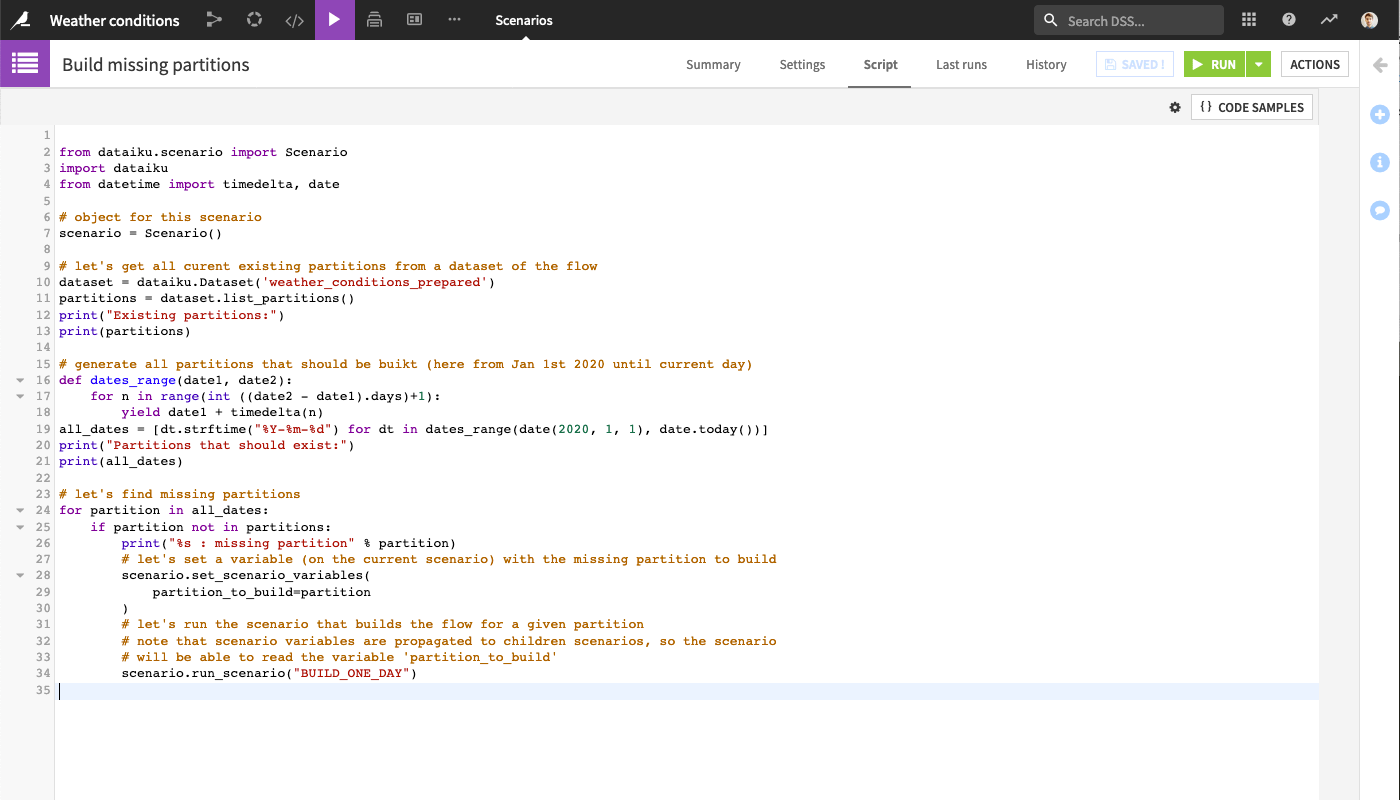Finally, you can run the scenario and see in the list of jobs that missing partitions get built.